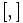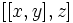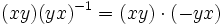# Proof of Baer construction of Lie group for Baer Lie ring

## Statement

This is a part of the Baer correspondence.

Suppose$L$ is a Baer Lie ring (?), i.e., a uniquely 2-divisible class two Lie ring, with addition denoted$+$ and Lie bracket denoted$[ , ]$. We give$L$ the structure of a 2-powered class two group as follows:

Group operation that we need to define Definition in terms of the Lie ring operations Further comments
Group multiplication$xy := x + y + \frac{1}{2}[x,y]$ Since center of uniquely 2-divisible Lie ring is uniquely 2-divisible, we obtain that the element$\frac{1}{2}[x,y]$ is central.
Identity element for multiplication Same as the zero element$0$ of the Lie ring.
Multiplicative inverse$x^{-1}$. Same as the additive inverse$-x$.
Group commutator$[x,y] = xyx^{-1}y^{-1}$ Same as the Lie bracket$[x,y]$.

## Proof

### Multiplication is associative

To prove:$\! (xy)z = x(yz)$

Proof: We have:$\! (xy)z = \left(x + y + \frac{1}{2}[x,y]\right) + z + \frac{1}{2}\left[x + y + \frac{1}{2}[x,y],z\right]$

We use that the linearity of the Lie bracket, and also use that the Lie ring has nilpotency class two to simplify$[[x,y],z]$ to zero. The expression simplifies to:$\! (xy)z = x + y + z + \frac{1}{2}\left([x,y] + [x,z] + [y,z]\right)$

Similarly, we can show that:$\! x(yz) = x + y + z + \frac{1}{2}\left([x,y] + [x,z] + [y,z]\right)$

Thus, associativity holds.

### Agreement of identity and inverses

Since$[x,0] = [0,x] = 0$, we have$x \cdot 0 = x + 0 + \frac{1}{2}[x,0] = x$, and similarly,$0 \cdot x = x$.

Further, since$[x,-x] = 0$, we have$x \cdot (-x) = x + (-x) + \frac{1}{2}[x,-x] = 0$, and similarly,$(-x) \cdot x = 0$.

### Commutator agrees with Lie bracket

To prove: The multiplicative commutator$[x,y] = (xy)(yx)^{-1}$ equals the Lie bracket$[x,y]$

Proof: We have:$(xy)(yx)^{-1} = (xy) \cdot (-yx)$

which becomes:$\! (xy)(yx)^{-1} = xy + (-yx) + \frac{1}{2}[xy,-yx]$

which simplifies to:$\! (xy)(yx)^{-1} = x + y + \frac{1}{2}[x,y] - (y + x + \frac{1}{2}[y,x]) + \frac{1}{2}[x + y + \frac{1}{2}[x,y],-(y + x + \frac{1}{2}[y,x])]$

Simplifying, and using the fact that the Lie ring has nilpotency class two (so applying a Lie bracket twice gives zero), we are left with the Lie bracket$[x,y]$.

### Class two

Since the commutator agrees with the Lie bracket, the class two condition on the Lie ring forces the class two condition on the Lie ring.

### 2-powered

The squaring map for the group operation agrees with the doubling map of the additive group of the Lie ring. Explicitly:$x^2 = x + x + \frac{1}{2}[x,x] = 2x$

We know that the Lie ring is 2-powered, i.e., the doubling map of the additive group of the Lie ring is bijective. Hence, the squaring map of the group is also bijective, i.e., the group is 2-powered.